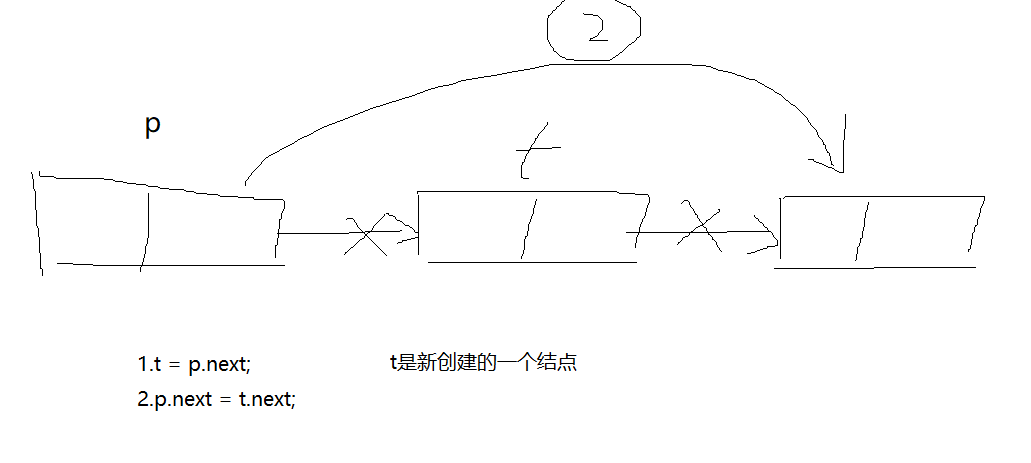数据结构（java版）学习笔记（三）——线性表之单链表

单链表的优点：

1. 长度不固定，可以任意增删。

单链表的缺点：

1. 存储密度小，因为每个数据元素，都需要额外存储一个指向下一元素的指针（双链表则需要两个指针）。

2. 要访问特定元素，只能从链表头开始，遍历到该元素，时间复杂度为 $O(n)$。在特定的数据元素之后插入或删除元素，不涉及到其他元素的移动，因此时间复杂度为 $O(1)$。双链表还允许在特定的数据元素之前插入或删除元素。

3. 存储空间不连续，数据元素之间使用指针相连，每个数据元素只能访问周围的一个元素（根据单链表还是双链表有所不同）。

单链表基本运算：

1.插入算法（核心）2.删除算法4.查找算法

Lnode t = h.next;
while(t!=null){
if(t.data==x){
System.out.println("找到");
return t;
}
t = t.next;
}
return null;

单链表的实现：

import java.util.Scanner;

Lnode h =null;
public static String toucha = "头插法";
public static String weicha = "尾插法";

//如果参数是头插法则使用头插法创建单链表，不是则使用尾插法
if(s.equals(toucha)){
h=new Lnode();
h.data = 'f';
h.next = null;
Lnode p;
Scanner scanner = new Scanner(System.in);
String str = scanner.nextLine();
for(int i=0;i<str.length();i++){

p = new Lnode();
p.data = str.charAt(i);

p.next = h.next;
h.next = p;
}
scanner.close();
}else{
h=new Lnode();
h.data = 'f';
h.next = null;

Lnode p,t;
t=h; //t用来代替头结点，同时，也就是
Scanner scanner = new Scanner(System.in);
String str = scanner.nextLine();
for(int i=0;i<str.length();i++){

p = new Lnode();
p.data = str.charAt(i); //接收String中的char

p.next = t.next;//此条语句与p.next =null 等同
t.next = p;
t = p; // t结点一直指向链表的末尾

}
scanner.close();
}
}
public void display(){
Lnode p = h.next;
while(p!=null){
System.out.println(p.data);
p = p.next;
}

}
/**
* 设置头结点
* @param _h
*/
public void setH(Lnode _h){
h=_h;
}
/**
*
* @param p 结点
* @param x 某个结点的值为x
* 将值为x的结点插入到p结点的后面
*/
public void insertElementAfter(Lnode p,char x){
Lnode t = new Lnode(x);
t.next = p.next; //这里容易忘记
p.next=t;
}
/**
*
* @param x
* @return 查找到值为x的结点
*/
public Lnode search(char x){
Lnode t = h.next;
while(t!=null){
if(t.data==x){
System.out.println("找到");
return t;
}
t = t.next;
}
return null;
}
@Override
public int size() {
int i =0;
Lnode t = h.next;
while(t!=null){
i++;
t = t.next;
}
return i;
}

@Override
public void clear() {
h.next = null;

}

@Override
public boolean isEmpty() {
if(h.next==null){
return false;
}else{
return true;
}
}
/**
*
* @param i 需要找到的第i个结点
* @return 第i个结点
*/
public Lnode getLnode(int i){
Lnode t = h; //从头结点算起，j就是从0开始
int j =0;
while(j<i){
t = t.next;
j++;
}
if(t==null){
return null;
}else{
return t;
}
}

@Override
public String get(int i) {
//取得第i个结点的值
Lnode t = h; //从头结点算起，j就是从0开始
int j =0;
while(j<i){
t = t.next;
j++;
}
//或者从第一个结点开始
/*Lnode t = h.next;
int j =1;
while(j<i){
t = t.next;
j++;
}*/
//这里需要加一个溢出处理
if(t==null){
return null;
}else{
return String.valueOf(t.data);
}

}

@Override
public int indexOf(String s) {
//单链表不需要复写此方法
return 0;
}

@Override
public String getPre(String s) {
//单链表不需要复写此方法
return null;
}

@Override
public String getNext(String s) {
// TODO Auto-generated method stub
return null;
}

@Override
public void insertElementAt(String s, int i) {
// TODO Auto-generated method stub

}

@Override
public String remove(int i) {
//先找到第i个结点，之后再将其移出,斌返回其的数值
Lnode t = getLnode(i);
Lnode q = getLnode(i-1); //q为t的前趋
q.next = t.next;

return String.valueOf(t.data);
}

@Override
public String remove(String s) {
// TODO Auto-generated method stub
return null;
}

}

补充一下：

next()一定要读取到有效字符后才可以结束输入，对输入有效字符之前遇到的空格键、Tab键或Enter键等结束符，next()方法会自动将其去掉，只有在输入有效字符之后，next()方法才将其后输入的空格键、Tab键或Enter键等视为分隔符或结束符。

简单地说，next()查找并返回来自此扫描器的下一个完整标记。完整标记的前后是与分隔模式匹配的输入信息，所以next方法不能得到带空格的字符串。

nextLine()方法的结束符只是Enter键，即nextLine()方法返回的是Enter键之前的所有字符，它是可以得到带空格的字符串的。

posted @ 2018-08-05 14:04  Stars-one  阅读(1158)  评论(0编辑  收藏  举报